## research papersBIOLOGICALCRYSTALLOGRAPHY
ISSN: 1399-0047
Volume 55| Part 10| October 1999| Pages 1759-1764

## Detecting outliers in non-redundant diffraction data

aDepartment of Haematology, University of Cambridge, Cambridge Institute for Medical Research, Wellcome Trust/MRC Building, Hills Road, Cambridge CB2 2XY, England
*Correspondence e-mail: rjr27@cam.ac.uk

(Received 18 May 1999; accepted 22 June 1999)

Outliers are observations which are very unlikely to be correct, as judged by independent observations or other prior information. Such unexpected observations are treated, effectively, as being more informative about possible models, so they can seriously impede the course of structure determination and refinement. The best way to detect and eliminate outliers is to collect highly redundant data, but it is not always possible to make multiple measurements of every reflection. For non-redundant data, the prior expectation given either by a Wilson distribution of intensities or model-based structure-factor probability distributions can be used to detect outliers. This captures mostly the excessively strong reflections, which dominate the features of electron-density maps or, even more so, Patterson maps. The outlier rejection tests have been implemented in a program, Outliar.

Keywords: Outliar; Outliers.

### 1. Introduction

When experimental data such as X-ray diffraction data are collected, the observations are subject to errors. As long as the sources of error are understood and properly accounted for, measurement errors do not cause serious problems; they just render the data less informative. However, some sources of error are either sporadic or are not properly accounted for. These include cosmic rays, zingers' in data collected with CCD detectors, and unmodelled shadows and dead areas on the detector surface. Such sources of error lead to rogue observations or outliers, which (if not detected) can cause great trouble.

The problem is that an outlier is an observation that is highly unlikely to occur, given one's understanding of the errors. The more unlikely an observation is, the more influence it has on the model developed to explain the data. This is particularly clear in the maximum-likelihood formalism, where the pressure to improve the fit to an observation depends precisely on the probability of having made the observation. So outliers can have a serious impact on the success of structure determination and refinement.

The standard, and still the best, way to cope with outliers is to collect highly redundant data. Outliers show up as single observations that agree very poorly with the bulk of other observations. Unfortunately, it is not always possible to collect highly redundant data, especially from crystals with low symmetry: synchrotron beam time is limited, crystals decay and equipment fails. Even in a highly redundant data set, there may still be some intensities that are only measured once or twice. When there are only two observations, and they disagree, some additional criterion is needed to adjudicate between them. For these reasons, it is desirable to have a means for detecting outliers without relying on redundancy.

### 2. What is an outlier?

Although outliers are typically detected by comparison with other observations in a redundant data set, an outlier is not just an observation that deviates from other observations. Random errors can be large and, as long as the understanding of the sources of errors is correct, the standard uncertainty (s.u.) will be large, and comparable to the size of deviations. If such an observation is merged with other observations, it will have an appropriate influence on the mean value, depending on the precision of other observations. Problems only arise when the error is much larger than one would expect from the s.u. Therefore, an outlier is an observation that is unlikely to be correct within error limits.

To test for an outlier, then, one needs to know something about the distribution of errors. Typically, the criterion for an outlier-rejection test is the probability of an observation deviating from its expected value by the amount observed or more. The application of this criterion can be illustrated easily for redundant observations with Gaussian measurement errors.

We divide the set of n observations into two groups: the observation we are testing and all the rest. The rest of the observations tell us what we would know about the true value without making the observation we are testing. If we assume Gaussian measurement errors, then the probability distribution for the true value, based on the n − 1 subset, is a Gaussian with a mean and standard uncertainty as found in standard textbooks on probability theory: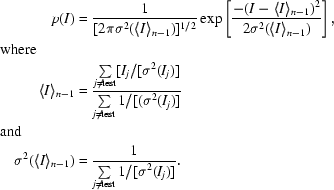Before we make the observation we are testing, we expect it to fall within this probability distribution smeared out additionally by the uncertainty introduced by a new measurement error.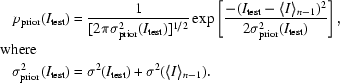To test a particular observation, we look at the probability that the observation could deviate that much or more from the expected value. Of course, since we are assuming a Gaussian error distribution, we can equivalently use a particular number of standard deviations from the mean as our criterion. In SCALA (Evans, 1993), a program to scale and merge diffraction data, the default is six standard deviations, which corresponds to about one chance in 109 of such a deviation arising by chance. More precisely, the probability of a positive deviation of this size or greater is 0.8 × 10−9. (A test like this, which looks for a deviation in only one direction, is called a one-tailed test.) The probability of a deviation of the same magnitude in the negative direction is the same, so the total probability of a deviation of that magnitude in either direction (two-tailed test) is 1.6 × 10−9.

In practice, one must consider the possibility that more than one of the redundant observations is an outlier, so in SCALA this procedure is carried out iteratively, testing each observation against the others and rejecting no more than one from a set at a time. Special criteria are used to decide which of only two observations should be accepted; in this situation, the statistical criteria described below for non-redundant observations could be used to adjudicate.

### 3. Structure-factor probabilities

If we have redundant data, each observation can be judged by how it compares with the other observations. We can think of the other observations as providing a prior expectation. However, if there is only a single observation, we have to obtain this prior expectation from another source. A possible source is structure-factor probabilities, i.e. what we know about possible values for the structure factor from prior information about the unit-cell content. The prior information can simply be that the cell contains a certain number of atoms in some more-or-less arbitrary arrangement, in which case we can use Wilson statistics (Wilson, 1949) to determine the probability of the observation. Alternatively, if we have an atomic model, we can use model-based probability distributions (Read, 1990). In either case, we determine the parameters for the probability distributions from the other reflections in the data set.

#### 3.1. Normalization

For both the Wilson and model-based outlier tests, it is convenient to work with normalized structure factors (E values), because one parameter (ΣN) is eliminated. Subsequent calculations are simplified if the expected value of E2 is unity for all classes of reflections. This requires accounting for the statistical effect of symmetry on intensity through the expected intensity factor, , which is the number of symmetry-related molecules that diffract systematically in phase for that class of reflection. If this factor is not taken into account, some legitimate observations will be rejected systematically from classes with larger values of . Data can readily be normalized by computing the Wilson parameter ΣN for resolution shells, as performed in the program SIGMAA (Read, 1986). If the resolution shells each contain 500–1000 reflections, the statistical error in estimates of ΣN is low and normalization is relatively insensitive to the presence of a few outliers,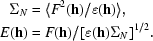### 4. Detecting outliers with Wilson statistics

The Wilson distribution of intensities can be computed from other structure factors in the same resolution shell, even if there are no redundant observations. In the Wilson distribution, weak intensities are very probable, so it is not useful for finding observations that are too small. However, as the intensity increases, the probability of making a measurement drops exponentially. So the Wilson distribution is useful for detecting and rejecting extremely large intensities such as those caused by cosmic rays and zingers'. As will be shown below, such outliers can be damaging to structure determination and refinement.

The test that is used is to compute the probability that an observation could be as large as or larger than the one made, i.e.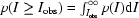. (This is a `one-tailed' test, as described above.) If one works with E values, this discriminator has a relatively simple form. For centric reflections,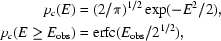where erfc is the complement of the error function. For acentric reflections,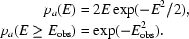This can be expressed in terms of normalized intensities, by a simple change of variables.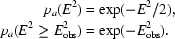Fig. 1illustrates the test for an acentric reflection. Since the test discriminators are only functions of the E values, the test can be implemented as a limit on maximum E value (with separate maxima for centric and acentric reflections). For instance, if one wished to reject observations as outliers if there were only one chance in a million of them arising by chance in the Wilson distribution, the limits on E would be about 3.72 for acentric reflections and 4.89 for centric reflections. For a probability of 10−9, the limits would be 4.55 (acentric) and 6.40 (centric).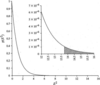Figure 1 Wilson distribution for acentric E2. The inset illustrates the integral defining the probability of an E2 of 13.82 (E = 3.72) or greater, which is about 10−6.

### 5. Detecting outliers using calculated structure factors

Near the end of a structure determination, additional restrictions can be placed on the structure factors by using prior probabilities derived from the calculated structure factors. Of course, to exploit this it is necessary to repeat the scaling and merging of the diffraction data near the end of refinement. Apart from tightening the restrictions on strong reflections, one can also detect observations that are too weak.

Appropriate probability distributions have been derived (Luzzati, 1952; Sim, 1959) and further generalized (Srinivasan, 1966; Read, 1990). When expressed in terms of normalized structure factors, they have a particularly simple form, depending only on a single parameter σA. This parameter can be thought of intuitively as the fraction of the calculated E value that is correct. The probability distribution is shown for the acentric case: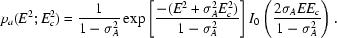Surprisingly, models must be fairly well refined to tighten the restrictions of the Wilson distribution significantly. Fig. 2illustrates sample probability curves for models with different values of σA. At a medium stage of resolution, σA values would typically be around 0.7, the curve for which looks very much like a Wilson distribution. Well refined models have values of σA that are not much above 0.95 in the medium-resolution data that agree best. As Fig. 2illustrates, a calculated structure factor will rarely provide as much information about the true structure factor as even a single weak experimental observation. On the other hand, when calculated E values are particularly large or small, the model-based distributions become more powerful in detecting outliers that are, respectively, too small or large.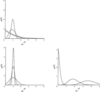Figure 2 Comparison of model-based probabilities with probability distributions from measurement error. (a) Model-based probability distributions for E2c  = 1 and σA values of 0, 0.7, 0.95 and 0.99. The distribution for a σA of zero is equivalent to a Wilson distribution, and as σA increases, the distributions become sharper. (b) Gaussian probability distributions for E2 = 1 and measurement s.u.s of 0.5, 0.2 and 0.1. In other terms, these correspond to 2σ, 5σ and 10σ measurements. (c) Model-based probability distributions for σA = 0.95 and E2c of 0.1, 2 and 4. Calculated structure factors at one extreme are more useful for detecting outliers at the other extreme.

### 6. Implementations of outlier detection and rejection

The algorithms described in this paper have been implemented in the program Outliar, which works with a merged data set in the form of a CCP4 MTZ file (Collaborative Computational Project, Number 4, 1994). This program reads in observed (and, optionally, calculated) structure factors. If calculated structure factors are provided, σA values needed for the structure-factor probabilities can be computed from the full data set or (preferably) cross-validation data, using an algorithm that has been briefly discussed in previous publications (Pannu & Read, 1996; Read, 1997). Both the Wilson distribution test and the model-based test, if applicable, are performed. The model-based test uses the MLF likelihood function (Pannu & Read, 1996), which is a Gaussian approximation that includes the effect of both model and measurement errors. If requested, an MTZ file omitting outliers can be written.

In principle, the presence of outliers could influence the estimates of ΣN and σA, which would imply that the outlier tests should be applied iteratively, with ΣN and σA being re-estimated between cycles. In practice, there are sufficient reflections in each resolution shell to minimize the impact of such effects.

Of course, it is more appropriate to remove outliers from the raw unmerged data. The test based on the Wilson distribution has been implemented by Phil Evans in the program SCALA (Evans, 1993), which scales and merges diffraction data. But for reasons discussed below, if this option is used on data from a crystal expected to display NCS, it would be best to use generous cutoff values to avoid rejecting legitimate reflections.

### 7. Impact of outliers on refinement

Outliers are observations that are extremely improbable or are unlikely to occur according to our understanding of the experiment and its sources of error. Because it is assumed in deriving likelihood targets for refinement that the error model is correct (Pannu & Read, 1996), outliers can have a serious impact on the quality of refinement. The log likelihood target is composed simply of the logs of the probabilities for each observation. An improbable observation will contribute a large negative number. In maximizing the likelihood, then, there will be great pressure to improve the agreement with outliers.

In maximum-likelihood structure refinement, as the model improves the expected size of model errors decreases and the probability distributions become sharper. Because of this, an outlier will have increasing impact on the progress of refinement as the refinement proceeds.

A test refinement was performed on the trypanosomal glycosomal triosephosphate isomerase (gTIM) to demonstrate the potential impact. In one region of reciprocal space, the 1.83 Å gTIM data set (Wierenga et al., 1991) has a number of outliers, which were detected using the program Outliar described below. (The author assisted in collecting this data set, which was kindly provided by Dr Rik Wierenga.) The largest E value in the set of 38819 data is 8.7; the probability of seeing a value at least that large is about 10−33, according to Wilson statistics. Using a cutoff of 10−6, 51 outliers were eliminated from the data set. Two parallel refinements were carried out in CNS (Brunger et al., 1998), differing only in whether these 51 reflections were rejected. The MLF target (Pannu & Read, 1996) was used for coordinate refinement, which was followed by restrained B-factor refinement and another round of coordinate refinement. The starting model was an intermediate model (Wierenga et al., 1987) refined against data to 2.4 Å before the high-resolution data were collected. The success of test refinements was judged by comparison with the final 1.83 Å model (Wierenga et al., 1991).

Fig. 3shows that the small number of outliers, only 0.13% of the entire data set, has a significant impact on the course of refinement. In addition, an inspection of the calculated structure factors from the two refined models shows that the refinement has indeed been skewed in the presence of the outliers; the average value of the calculated structure factors for these 51 reflections is 2.95 times as large when the refinement is carried out including the outliers. As argued above, one would expect these outliers to have an increasing impact towards the end of refinement, as they become even more improbable.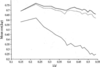Figure 3 Effect of outliers on refinement of gTIM. Model quality is judged by the mean cosine of the phase difference from the final refined model (Wierenga et al., 1991). Results are shown for the starting model (thin line), the model after refinement including outliers (dashed line) and the model after refinement excluding outliers (thick line).

### 8. Future developments

As it is currently implemented, the outlier-rejection algorithm runs the risk of rejecting legitimate observations that are subject to effects that have not yet been modelled. One major problem is that the normalization scheme for the determination of E values used in the rejection test based on the Wilson distribution assumes that the falloff of the diffraction pattern is isotropic and can be modelled by a resolution-dependent curve. Unfortunately, many crystals diffract anisotropically and reflections from the directions that diffract strongly could end up being discarded. The workaround that can be applied is to scale the data anisotropically to remove the anisotropic component of falloff before applying the rejection test, but it would be better to model anisotropic diffraction explicitly.

The second major problem is that the statistical effect of non-crystallographic symmetry (NCS), particularly translational NCS, has not yet been accounted for. As discussed above, it is essential to account for the effect of crystallographic symmetry through the expected intensity factor to avoid rejecting reflections from certain classes systematically. Similarly, NCS can modulate the expected intensities. Most seriously, translational NCS can lead to certain reflections being increased in their expected intensity by a factor equal to the number of similarly oriented molecules. Until these effects are accounted for, it will be important to use very relaxed criteria for the rejection of outliers in data sets from crystals with translational NCS.

Outlier rejection in SCALA (or, from a reduced data set, in Outliar) can eliminate at least the worst rogue observations. However, as discussed above, the information in the calculated structure factor comes to place a restraint on possible values of the observed structure factor, especially towards the end of refinement. If reflections that come to be seen as improbable are used in refinement, they will have an inordinate effect on the course of refinement. It should be possible to also implement outlier-detection algorithms in refinement programs, where they could be used to automatically downweight suspect observations in a robust/resistant refinement procedure.

Finally, the same relationship that exists between the true structure and a model exists between a native protein and an isomorphous derivative (or ligand-bound species). Therefore, the model-based outlier-detection algorithm could also be used to detect improbable pairs of structure factors in heavy-atom/native or ligand-bound/native pairs of observations. For heavy-atom derivatives, in particular, this statistical test could be quite important, as the deviations are squared when computing difference Patterson maps.

### Acknowledgements

Bart Hazes, Navraj Pannu and Phil Evans took part in discussions that helped to clarify the ideas presented in this paper. Rik Wierenga generously supplied the data used in test calculations. This research was supported by the Wellcome Trust (award 050211).

### ReferencesBIOLOGICALCRYSTALLOGRAPHY